# The MDC Procedure

### Model Fit and Goodness-of-Fit Statistics

McFadden (1974) suggests a likelihood ratio index that is analogous to the R-square in the linear regression model: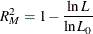where
is the maximum of the log-likelihood function and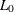is the maximum of the log-likelihood function when all coefficients, except for an intercept term, are zero. McFadden’s likelihood ratio index is bounded by 0 and 1.

Estrella (1998) proposes the following requirements for a goodness-of-fit measure to be desirable in discrete choice modeling:

• The measure must take values in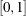, where 0 represents no fit and 1 corresponds to perfect fit.

• The measure should be directly related to the valid test statistic for the significance of all slope coefficients.

• The derivative of the measure with respect to the test statistic should comply with corresponding derivatives in a linear regression.

Estrella’s measure is written as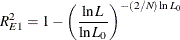Estrella suggests an alternative measure,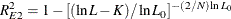where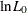is computed with null parameter values,
is the number of observations used, and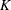represents the number of estimated parameters.

Other goodness-of-fit measures are summarized as follows: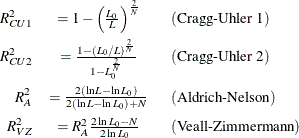The AIC and SBC are computed as follows: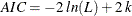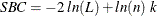where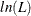is the log-likelihood value for the model, k is the number of parameters estimated, and n is the number of observations (that is, the number of respondents).